Powers of Trigonometric FunctionsCustom SearchPOWERS OF TRIGONOMETRIC FUNCTIONS The integrals of powers of trigonometric functions will be limited to those which may, by substitution, be written in the form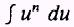EXAMPLE: Evaluate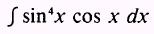SOLUTION: Letso thatBy substitution,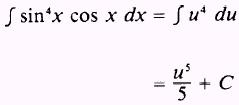Then, by substitution again, find that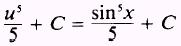Therefore,EXAMPLE: Evaluate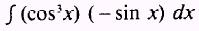SOLUTION: Let u = cos x so that du = -sin x dx We know that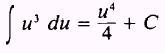so by substitution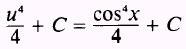PRACTICE PROBLEMS: Evaluate the following integrals: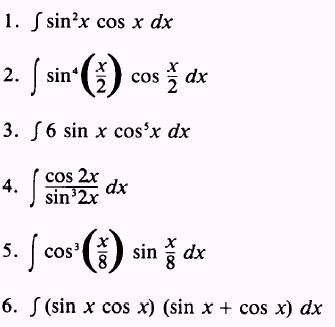ANSWERS:SUMMARY The following are the major topics covered in this chapter: 1. Integral of a variable to a power: The integral of a variable to a power is the variable to a power increased by one and divided by the new power. Formula.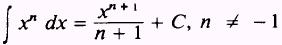2. Integral of a constant: A constant may be written either before or after the integral sign. Formula.3. Integral of the sum of differentiable functions: The integral of an algebraic sum of differentiable functions is the same as the algebraic sum of the integrals of these functions taken separately. Formula.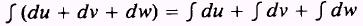4. Integral of a function raised to a power: The integral of a function raised to a power is found by the following steps: 1. Increase the power of the function by 1. 2. Divide the result of step 1 by this increased power. 3. Add the constant of integration. Formula.5. Integral of quotients: Method 1. Integrate by putting the quotient into the form of the power of a function. Method 2. Integrate quotients by use of operations of logarithms. Formula.Method 3. Integrate quotients by changing the integrand into a polynomial plus a fraction by dividing the denominator into the numerator. 6. Integral of a constant to a variable power: Formula.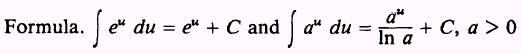where u is a variable, ais any constant, and e is a defined constant. 7. Integral of trigonometric functions: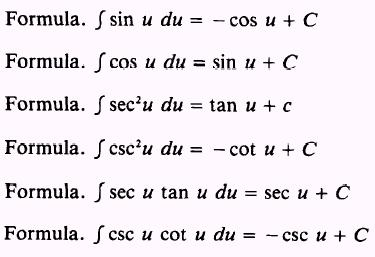8. Integral of powers of trigonometric functions: The integrals of powers of trigonometric functions will be limited to those which may, by substitution, be written in the form J u" du. ADDITIONAL PRACTICE PROBLEMS Evaluate the following integrals: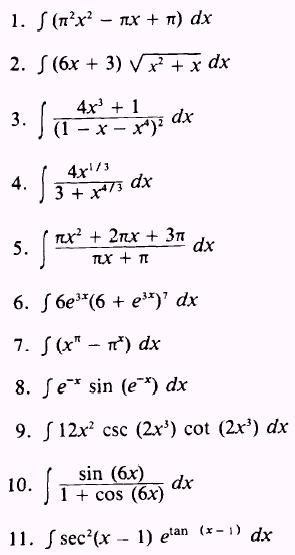ANSWERS TO ADDITIONAL PRACTICE PROBLEMSIntegrated Publishing, Inc. - A (SDVOSB) Service Disabled Veteran Owned Small Business